6.041SC | Fall 2013 | Undergraduate
Probabilistic Systems Analysis and Applied Probability

## Unit IV: Laws Of Large Numbers And Inference

« Previous | Next »

In this section, we start with a discussion of limit theorems: the weak law of large numbers and the central limit theorem. We then introduce the subject of inference (estimation and hypothesis testing), from two alternative viewpoints: first, Bayesian inference, which relies on a prior distribution for unknown quantities and on the Bayes rule to incorporate new evidence; and, second, classical inference, in which no probabilistic assumptions are made on the unknown quantities and instead relies heavily on the laws of large numbers to provide statistical guarantees, e.g., in the form of confidence intervals.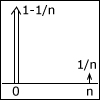Lecture 19: Weak Law of Large Numbers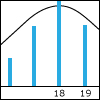Lecture 20: Central Limit Theorem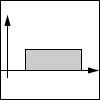Lecture 21: Bayesian Statistical Inference - I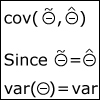Lecture 22: Bayesian Statistical Inference - II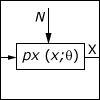Lecture 23 Classical Statistical Inference - I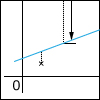Lecture 24: Classical Inference - II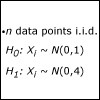Lecture 25: Classical Inference - III

Looking for something specific in this course? The Resource Index compiles links to most course resources in a single page.

« Previous | Next »